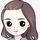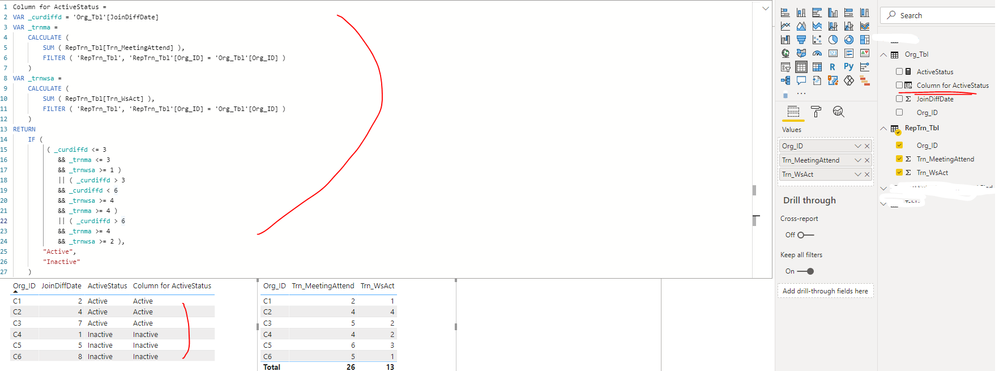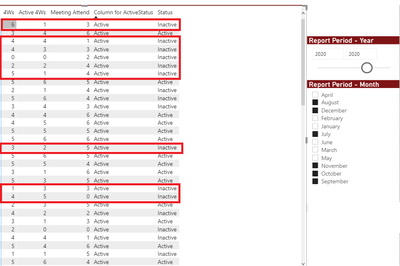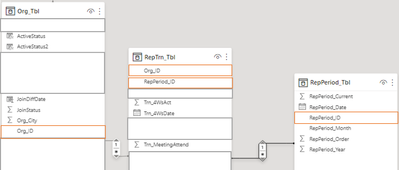cancel
Showing results for
Did you mean:

Fabric is Generally Available. Browse Fabric Presentations. Work towards your Fabric certification with the Cloud Skills Challenge.Helper I

## Group by and Conditions

Dear Community Members,

I have two Queries, called: Org_Tbl, RepTrn_Tbl. Org_tbl has the customers' names, while the RepTrn_Tbl has the attendances and reports information. It has a one to money relation. I want to create a column to check if these customers are active or not in past period, where I have athird Query record the attendance and report dates.

It is something such as this IF statement:

``ActiveStatus = IF(Org_Tbl[JoinDiffDate]<=3 && SUM(RepTrn_Tbl[Trn_MeetingAttend])<=3 && SUM(RepTrn_Tbl[Trn_WsAct])>=1,"Active",IF(Org_Tbl[JoinDiffDate] > 3 && Org_Tbl[JoinDiffDate] < 6 && SUM(RepTrn_Tbl[Trn_WsAct])>=4 && SUM(RepTrn_Tbl[Trn_MeetingAttend])>=4,"Active",IF(Org_Tbl[JoinDiffDate] > 6 && SUM(RepTrn_Tbl[Trn_MeetingAttend]) >= 4 && SUM(RepTrn_Tbl[Trn_WsAct])>=2,"Active","Inactive")))``

I know it has to group by RepTrn_Tbl[Org_ID], but I am new to DAX and I could not find the solution. Any other suggestion, appreciated!.

Best Regards

Mahmoud

2 ACCEPTED SOLUTIONSCommunity Support

Hi @mahmoud ,

In fact, , I created a measure rather than a calculated column in my previous post. If you need to create a calculated column, you can write it like this:

``````Column for ActiveStatus =
VAR _curdiffd = 'Org_Tbl'[JoinDiffDate]
VAR _trnma =
CALCULATE (
SUM ( RepTrn_Tbl[Trn_MeetingAttend] ),
FILTER ( 'RepTrn_Tbl', 'RepTrn_Tbl'[Org_ID] = 'Org_Tbl'[Org_ID] )
)
VAR _trnwsa =
CALCULATE (
SUM ( RepTrn_Tbl[Trn_WsAct] ),
FILTER ( 'RepTrn_Tbl', 'RepTrn_Tbl'[Org_ID] = 'Org_Tbl'[Org_ID] )
)
RETURN
IF (
( _curdiffd <= 3
&& _trnma <= 3
&& _trnwsa >= 1 )
|| ( _curdiffd > 3
&& _curdiffd < 6
&& _trnwsa >= 4
&& _trnma >= 4 )
|| ( _curdiffd > 6
&& _trnma >= 4
&& _trnwsa >= 2 ),
"Active",
"Inactive"
)``````Best Regards

Community Support Team _ Rena
If this post helps, then please consider Accept it as the solution to help the other members find it more quickly.Community Support

Hi @mahmoud ,

As checked your screenshot, there are some slicers applied in your report page. The value of a calculate column is computed during data refresh, it will not change by the user interaction in the report dynamically. So please create a measure instead of calculated column.

Calculated Columns and Measures in DAX

``````New measure for ActiveStatus =
VAR _curdiffd =
SELECTEDVALUE ( 'Org_Tbl'[JoinDiffDate] )
VAR _trnma =
CALCULATE (
SUM ( RepTrn_Tbl[Trn_MeetingAttend] ),
FILTER (
'RepTrn_Tbl',
'RepTrn_Tbl'[Org_ID] = SELECTEDVALUE ( 'Org_Tbl'[Org_ID] )
)
)
VAR _trnwsa =
CALCULATE (
SUM ( RepTrn_Tbl[Trn_4WsAct] ),
FILTER (
'RepTrn_Tbl',
'RepTrn_Tbl'[Org_ID] = SELECTEDVALUE ( 'Org_Tbl'[Org_ID] )
)
)
VAR _trnws =
CALCULATE (
SUM ( RepTrn_Tbl[Trn_4Ws] ),
FILTER (
'RepTrn_Tbl',
'RepTrn_Tbl'[Org_ID] = SELECTEDVALUE ( 'Org_Tbl'[Org_ID] )
)
)
RETURN
IF (
( _curdiffd <= 3
&& _trnws <= 3
&& _trnma <= 3
&& _trnwsa >= 1 )
|| ( _curdiffd > 3
&& _curdiffd < 6
&& _trnwsa >= 4
&& _trnma >= 4 )
|| ( _curdiffd > 6
&& _trnws >= 4
&& _trnma >= 4
&& _trnwsa >= 2 ),
"Active",
"Inactive"
)``````

Best Regards

Community Support Team _ Rena
If this post helps, then please consider Accept it as the solution to help the other members find it more quickly.
5 REPLIES 5Helper I

Hi @v-yiruan-msft , Hi @v-yingjl

I hope you are doing well,

I am sorry for getting back to you late, I was off in the past days.

Thanks for your support and respond, the new solution worked partially, as you see in the following image.

Actually, an extra condition I did not mention it, as guess the filter control will did it. The extra condition is to apply these rules on organization for past six months.

Here is updated code I used

``````Column for ActiveStatus New =
VAR _curdiffd = 'Org_Tbl'[JoinDiffDate]
VAR _trnma =
CALCULATE (
SUM ( RepTrn_Tbl[Trn_MeetingAttend] ),
FILTER ( 'RepTrn_Tbl', 'RepTrn_Tbl'[Org_ID] = 'Org_Tbl'[Org_ID] )
)
VAR _trnwsa =
CALCULATE (
SUM ( RepTrn_Tbl[Trn_4WsAct] ),
FILTER ( 'RepTrn_Tbl', 'RepTrn_Tbl'[Org_ID] = 'Org_Tbl'[Org_ID] )
)
VAR _trnws =
CALCULATE (
SUM ( RepTrn_Tbl[Trn_4Ws] ),
FILTER ( 'RepTrn_Tbl', 'RepTrn_Tbl'[Org_ID] = 'Org_Tbl'[Org_ID] )
)
RETURN
IF (
(     _curdiffd <= 3
&& _trnws <= 3
&& _trnma <= 3
&& _trnwsa >= 1 )
|| ( _curdiffd > 3
&& _curdiffd < 6
&& _trnwsa >= 4
&& _trnma >= 4 )
|| (
_curdiffd > 6
&& _trnws >= 4
&& _trnma >= 4
&& _trnwsa >= 2 ),
"Active",
"Inactive"
)``````Best Regards

MahmoudCommunity Support

Hi @mahmoud ,

As checked your screenshot, there are some slicers applied in your report page. The value of a calculate column is computed during data refresh, it will not change by the user interaction in the report dynamically. So please create a measure instead of calculated column.

Calculated Columns and Measures in DAX

``````New measure for ActiveStatus =
VAR _curdiffd =
SELECTEDVALUE ( 'Org_Tbl'[JoinDiffDate] )
VAR _trnma =
CALCULATE (
SUM ( RepTrn_Tbl[Trn_MeetingAttend] ),
FILTER (
'RepTrn_Tbl',
'RepTrn_Tbl'[Org_ID] = SELECTEDVALUE ( 'Org_Tbl'[Org_ID] )
)
)
VAR _trnwsa =
CALCULATE (
SUM ( RepTrn_Tbl[Trn_4WsAct] ),
FILTER (
'RepTrn_Tbl',
'RepTrn_Tbl'[Org_ID] = SELECTEDVALUE ( 'Org_Tbl'[Org_ID] )
)
)
VAR _trnws =
CALCULATE (
SUM ( RepTrn_Tbl[Trn_4Ws] ),
FILTER (
'RepTrn_Tbl',
'RepTrn_Tbl'[Org_ID] = SELECTEDVALUE ( 'Org_Tbl'[Org_ID] )
)
)
RETURN
IF (
( _curdiffd <= 3
&& _trnws <= 3
&& _trnma <= 3
&& _trnwsa >= 1 )
|| ( _curdiffd > 3
&& _curdiffd < 6
&& _trnwsa >= 4
&& _trnma >= 4 )
|| ( _curdiffd > 6
&& _trnws >= 4
&& _trnma >= 4
&& _trnwsa >= 2 ),
"Active",
"Inactive"
)``````

Best Regards

Community Support Team _ Rena
If this post helps, then please consider Accept it as the solution to help the other members find it more quickly.Community Support

Hi @mahmoud ,

Which column be based on when create relationship between these two table? Customer name field? If yes, please create a measure as below:

``````ActiveStatus =
VAR _curdiffd =
SUM ( 'Org_Tbl'[JoinDiffDate] )
RETURN
IF (
(
_curdiffd <= 3
&& SUM ( RepTrn_Tbl[Trn_MeetingAttend] ) <= 3
&& SUM ( RepTrn_Tbl[Trn_WsAct] ) >= 1
)
|| (
_curdiffd > 3
&& _curdiffd < 6
&& SUM ( RepTrn_Tbl[Trn_WsAct] ) >= 4
&& SUM ( RepTrn_Tbl[Trn_MeetingAttend] ) >= 4
)
|| (
_curdiffd > 6
&& SUM ( RepTrn_Tbl[Trn_MeetingAttend] ) >= 4
&& SUM ( RepTrn_Tbl[Trn_WsAct] ) >= 2
),
"Active",
"Inactive"
)``````

If the above one is not working in your scenario, please provide some sample data(exclude sensitive data) in Org_Tbl and RepTrn_Tbl table and the calculation logic about ActiveStatus. Thank you.

Best Regards

Community Support Team _ Rena
If this post helps, then please consider Accept it as the solution to help the other members find it more quickly.Helper I

Dear @v-yiruan-msft thanks for your support!

Kindly find in the following image the realtions bewtween the three tables.The logice you wrote for the IF statement is totally correct except the SUM of JoinDiffDate, this is a number to measure how long the organization has been registered

Best Regards

MahmoudCommunity Support

Hi @mahmoud ,

In fact, , I created a measure rather than a calculated column in my previous post. If you need to create a calculated column, you can write it like this:

``````Column for ActiveStatus =
VAR _curdiffd = 'Org_Tbl'[JoinDiffDate]
VAR _trnma =
CALCULATE (
SUM ( RepTrn_Tbl[Trn_MeetingAttend] ),
FILTER ( 'RepTrn_Tbl', 'RepTrn_Tbl'[Org_ID] = 'Org_Tbl'[Org_ID] )
)
VAR _trnwsa =
CALCULATE (
SUM ( RepTrn_Tbl[Trn_WsAct] ),
FILTER ( 'RepTrn_Tbl', 'RepTrn_Tbl'[Org_ID] = 'Org_Tbl'[Org_ID] )
)
RETURN
IF (
( _curdiffd <= 3
&& _trnma <= 3
&& _trnwsa >= 1 )
|| ( _curdiffd > 3
&& _curdiffd < 6
&& _trnwsa >= 4
&& _trnma >= 4 )
|| ( _curdiffd > 6
&& _trnma >= 4
&& _trnwsa >= 2 ),
"Active",
"Inactive"
)``````Best Regards

Community Support Team _ Rena
If this post helps, then please consider Accept it as the solution to help the other members find it more quickly.Announcements#### Power BI Monthly Update - November 2023

Check out the November 2023 Power BI update to learn about new features.#### Fabric Community News unified experience

Read the latest Fabric Community announcements, including updates on Power BI, Synapse, Data Factory and Data Activator.#### The largest Power BI and Fabric virtual conference

130+ sessions, 130+ speakers, Product managers, MVPs, and experts. All about Power BI and Fabric. Attend online or watch the recordings.Top Solution Authors
Top Kudoed Authors
Users online (3,667)